# ImageDIG 2.0.7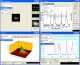ImageDIG consists of three integrated modules which allow the user to convert 2D flat images into 3D (x,y,z) data or if a graph, convert the graph in image form into 2 dimensional numerical data.

 Developer: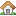SciCepts Engineering      software by SciCepts Engineering → Price: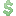89.00  buy → License: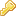Shareware File size: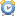0K Language: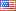OS: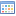Windows Vista (?) Rating: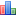0 /5 (0 votes)

ImageDIG consists of three integrated modules which allow the user to convert 2D flat images into 3D (x,y,z) data or if a graph, convert the graph in image form into 2 dimensional numerical data.

The third module is a full analysis plotting utility where the user can import either the 2D or 3D data saved above or import any number of columns of data from a file. The data can then be analyzed using several statistical functions and plotted.

ImageDIG was developed because other functionally similar programs (2D) either had a steep learning curve, incomplete or confusing to use. However, no other program includes an image to 3D numerical data converter and a general purpose x/y plotter.

A variety of data analysis options are available in this module. Example images for tutorial purposes are supplied. This includes real world images of an electron beam for the 3D digitizer.

Computer generated plots (linear, log, fractional, etc), scanned images from articles for the 2D digitizer, and ascii data files for the universal plotter.

Graph processing functions include statistical analysis such as: Min/Max/Mean Fitting - Standard deviation, best fit, and curve fit. Curve fit includes: variable-order polynomial, logarithmic, exponential, power, inverse, spline, and moving averages

Additional features include a built in bitmap editor, magnifier and spreadsheet style data viewer/editor for the 2D scanner module.
Complete control of the graph windows such as Labels, Legends, Grids, Colors, etc through the Graph Toolbox.

Import image from the clipboard or from a TWAIN compatible device directly into the 2D or 3D scanner image box including Web images.

tags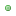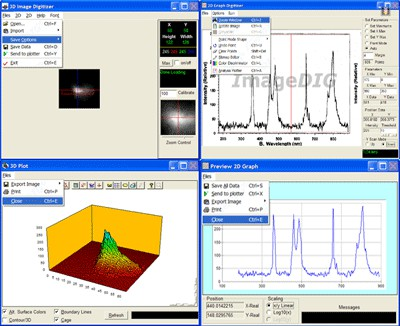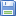Download ImageDIG 2.0.7

Purchase: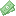Buy ImageDIG 2.0.7

###### Authors softwareImageDIG 2.0.7
SciCepts Engineering

ImageDIG consists of three integrated modules which allow the user to convert 2D flat images into 3D (x,y,z) data or if a graph, convert the graph in image form into 2 dimensional numerical data.

###### Similar softwareImageDIG 2.0.7
SciCepts Engineering

ImageDIG consists of three integrated modules which allow the user to convert 2D flat images into 3D (x,y,z) data or if a graph, convert the graph in image form into 2 dimensional numerical data.Nonlinear regression analysis - CurveFitter 1.1
Institute of Mathematics and Statistics

Nonlinear regression analysis - CurveFitter software performs statistical regression analysis to estimate the values of parameters for linear, multivariate, polynomial, exponential and nonlinear functions.LAB Fit Curve Fitting Software 7.2.38
Wilton Pereira da Silva

LAB Fit Curve Fitting is an application that can help with the process and analysis of experimental data.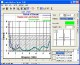Graph Digitizer Scout 1.24
ByteScout

Graph Digitizer Scout will automatically convert hard-copy graphs, oscillograms, charts, plots, into X,Y.GetData Graph Digitizer 2.22
S. Fedorov

GetData Graph Digitizer is a software that lets you digitize graphs and plots.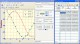Rt-Plot 2.8.10.83
Rt-Science

Rt-Plot is a useful tool that will generate Cartesian X/Y-plots from scientific data.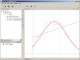GraphSight 2.0.1

GraphSight is designed for 2D math graphing needs.xyExtract Graph Digitizer 2.4
Wilton Pereira da Silva

The "xyExtract" software is used for to extract data from a 2D graph (orthogonal and nonorthogonal axes) contained in a graphic file (scanned, PDF document or in a some file like gif, jpg, etc).Graph Plotter 1.2.3
Still Soft

Graph Plotter is a powerful utility that will help you plot and analyze different types of graphs.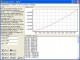Equation graph plotter - EqPlot 1.2
Institute of Mathematics and Statistics

Equation graph plotter software plots 2D graphs from complex mathematical equations.

###### Other software in this category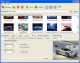AnyToJpeg 3.0
PhotoActions

There are a lot of graphic editors.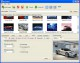AnyToGif 2.0
PhotoActions

There are a lot of graphic editors.Aeon Batch Image Editor 1.0
iRedSoft Technology Inc

Aeon Batch Image Editor is a Batch Image Processor for Webmasters and Digital Photographer.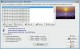Image Thumbnailer and Converter 2.36f
SoftDD

Image Thumbnailer and Converter allows you to create thumbnails, convert images, and view your photos an entire folder at a time.WaveL CompressIt 1.5
WaveL Software

WaveL CompressIt is an utility that allows you to select the most effective compression format for storing your image files.